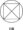# Object Oriented Programming July-2011

UNIT – I

1. (a) What are the different features of OOPS ?

(b) Differentiate C++ and JAVA.

(c) What is the use of virtual key word in multi path inheritance. Explain with the help of suitable example.

OR

(a) Define class and object with example and explain different access specifier.

(b) What are the advantages of OOPS ? Explain them.

UNIT – II

2 (a) Write a program to find maximum of two data/ value using friend function.

(b) Explain constructor and destructor with example.

OR

2. (a) Write a program to find area of rectangle, circle and square using function overloading.

(b) Explain virtual function and inline function with the help of example.

UNIT – III

3. (a) Wrhat is applet ? Explain life cycle of applet.

(b) Write a program in java using applet to draw the following figure.3 (a) Explain different types of variable in java and also JVM.

(b) Explain the following :

(i) JIT

(ii) Byte code

UNIT – IV

4.(a) Write a program in java to find inverse of a matrix.

OR

(a) Write a program to sort an integer array in decending order.

(b) Explain different operator used in java with the help of a program.

UNIT – V

5.(a) What is interface ? How you will create a interface ? Write all steps to create and implement a interface. How it is different with class ?

OR

(a)  Explain access protection in java.

(b)  How to import a package in class.